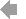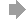Basic HTML VersionView Full Version
Page 220 - Course_Catalog_2020-2021
P. 220

``````MATH 23 Engineering Statistics
(See general education pages for the requirement this course meets.) Prerequisite: MATH 1C or MATH 1CH (with a grade of C or better). Advisory: EWRT 211 and READ 211, or ESL 272 and 273.
Five hours lecture (60 hours total per quarter).
5 Units
MATH 42 Precalculus II: Trigonometric Functions 5 Units
(See general education pages for the requirement this course meets.)
(Not open to students in MATH 42H.)
Prerequisite: MATH 41 or MATH 41H (with a grade of C or better); or a satisfactory score on the College Level Math Placement Test within the last calendar year.
Advisory: EWRT 211 and READ 211, or ESL 272 and 273. Five hours lecture (60 hours total per quarter).
The theory of trigonometric functions and their applications.
MATH 42H Precalculus II: Trigonometric Functions - 5 Units HONORS
(See general education pages for the requirement this course meets.)
(Not open to students with credit in MATH 42.)
(Admission into this course requires consent of the Honors Program Coordinator.) Prerequisite: MATH 41 or MATH 41H (with a grade of C or better); or a satisfactory score on the College Level Math Placement Test within the last calendar year.
Five hours lecture (60 hours total per quarter).
The theory of trigonometric functions and their applications. As an honors course, students will be expected to complete extra assignments to gain deeper insight in precalculus.
MATH 43 Precalculus III: Advanced Topics 5 Units
(See general education pages for the requirement this course meets.)
(Not open to students with credit in MATH 43H.)
Prerequisite: MATH 41 (or MATH 41H) and MATH 42 (or MATH 42H) (both with a grade of C or better); or a satisfactory score on Calculus Readiness Test within the last calendar year.
Five hours lecture (60 hours total per quarter).
Hyperbolic functions, parametric equations, systems of equations and inequalities, vectors, lines and planes, sequences and series, polar coordinates, mathematical induction, and the binomial theorem.
MATH 43H Precalculus III: Advanced Topics - 5 Units HONORS
(See general education pages for the requirement this course meets.)
(Not open to students with credit in MATH 43.)
(Admission into this course requires consent of the Honors Program Coordinator.) Prerequisite: MATH 41 (or MATH 41H) and MATH 42 (or MATH 42H) (both with a grade of C or better); or a satisfactory score on Calculus Readiness Test within the last calendar year.
Five hours lecture (60 hours total per quarter).
Hyperbolic functions, parametric equations, systems of equations and inequalities, vectors, lines and planes, sequences and series, polar coordinates, mathematical induction, and the binomial theorem. As an honors course, students will be expected to complete extra assignments to gain deeper insight in precalculus.
MATH 44 Mathematics in Art, Culture, and 5 Units Society: A Liberal Arts Math Class
(See general education pages for the requirement this course meets.) Prerequisite: Intermediate Algebra (MATH 109, MATH 114 or MATH 130)
or equivalent.
Five hours lecture (60 hours total per quarter).
This course is a survey of selected topics from contemporary mathematics, including problem-solving techniques and connections between mathematics and culture. It includes a selection of introductory topics from symmetry; graph theory; chaos and fractals; topology; number theory; geometry; combinatorics and counting; the mathematics of social choice; data analysis, probability, and statistics; consumer mathematics and personal financial management.
MATH 46 Mathematics for Elementary Education 5 Units
(See general education pages for the requirement this course meets.) Prerequisite: MATH 114 with a grade of C or better, or a qualifying score on Intermediate Algebra Placement Test within the past calendar year. Advisory: EWRT 211 and READ 211, or ESL 272 and 273.
(Also listed as EDUC 46. Students may enroll in either department, but not both, for credit.)
Five hours lecture (60 hours total per quarter).
Designed for prospective elementary and middle school teachers. An introduction to the discipline of mathematics as the use of logical, quantitative, and spatial reasoning in the abstraction, modeling, and problem solving of real-world situations. The main topics in the course include the origins of mathematics, mathematical reasoning and problem solving strategies, theory of sets, integers and integral number theory, rational numbers and proportion, real numbers and decimal notation, and measurement. Throughout the course students will experience the learning of mathematics in a way that models how they can create an active learning environment for their future students.
M
Engineering statistics provides a comprehensive introduction to probabilistic and statistical modeling for students in engineering, economics, finance and related disciplines in the mathematical sciences. The course exposes students to a variety of applications requiring decision making in the face of uncertainty. Topics covered include the collection and analysis of information, making use of graphical and numerical techniques, discrete, continuous, cumulative, and joint probability distribution functions and use of statistical inference, experimental design, and equation fitting, when appropriate. Many of the applications require the use of technology (computers and graphic calculators). Computer simulations are used to illustrate difficult topics and provide visualization of advanced theoretical results (e.g. the Central Limit Theorem).
MATH 31 Precalculus I
5 Units
(See general education pages for the requirement this course meets.) Prerequisite: Intermediate Algebra (MATH 109, MATH 114 or MATH 130)
or equivalent.
Five hours lecture (60 hours total per quarter).
This course covers polynomial, rational, exponential and logarithmic functions, graphs, solving equations, conic sections, systems of equations and inequalities, sequences and series.
MATH 31A Precalculus I (Part 1) 2 1/2 Units
(See general education pages for the requirement this course meets.) Prerequisite: Intermediate Algebra (MATH 109, MATH 114 or MATH 130)
or equivalent.
Corequisite: MATH 231A.
Two and one-half hours lecture (30 hours total per quarter).
This course covers linear, quadratic, power, absolute value, piecewise functions, graphs, solving equations and inequalities, solving systems of equations and inequalities.
MATH 31B Precalculus I (Part 2) 2 1/2 Units
(See general education pages for the requirement this course meets.) Prerequisite: MATH 31A (with a grade of C or better).
Corequisite: MATH 231B.
Two and one-half hours lecture (30 hours total per quarter).
Polynomial, rational, exponential and logarithmic functions, graphs, solving equations, conic sections.
MATH 32 Precalculus II 5 Units
(See general education pages for the requirement this course meets.) Prerequisite: MATH 31 or MATH 31B (with a grade of C or better); or a satisfactory score on college placement.
Five hours lecture (60 hours total per quarter).
Preparation for calculus: extending the elementary functions of first quarter precalculus to include the theory of periodic functions; composition of trigonometric functions with other elementary functions; polar co-ordinates; further exploration of the complex plane; introduction to the algebra of vectors.
MATH 41 Precalculus I: Theory of Functions
5 Units
(See general education pages for the requirement this course meets.)
(Not open to students with credit in MATH 41H.)
Prerequisite: Intermediate Algebra (MATH 114, MATH 109, MATH 130) or equivalent. Advisory: EWRT 211 and READ 211, or ESL 272 and 273.
Five hours lecture (60 hours total per quarter).
This course covers polynomial, rational, exponential and logarithmic functions, graphs, solving equations, conic sections.
MATH 41H Precalculus I: Theory of Functions - HONORS
5 Units
(See general education pages for the requirement this course meets.)
(Not open to students with credit in MATH 41.)
(Admission into this course requires consent of the Honors Program Coordinator.) Prerequisite: Intermediate Algebra (MATH 114, MATH 109, MATH 130) or equivalent. Advisory: EWRT 211 and READ 211, or ESL 272 and 273.
Five hours lecture (60 hours total per quarter).
This course covers polynomial, rational, exponential and logarithmic functions, graphs, solving equations, conic sections. Because it is an honors course, students will be expected to complete extra assignments to gain a deeper insight into precalculus.
2O20-2O21 DE ANZA COLLEGE CATALOG
219
All courses are for unit credit and apply to a De Anza associate degree unless otherwise noted.

``````218   219   220   221   222